Next: Alternative forms of Lagrange Up: Derivation of Lagrange planetary Previous: Transformation of Lagrange brackets

# Lagrange planetary equations

Now,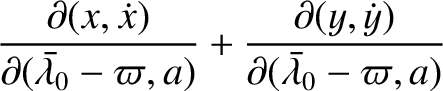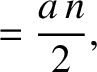(G.86)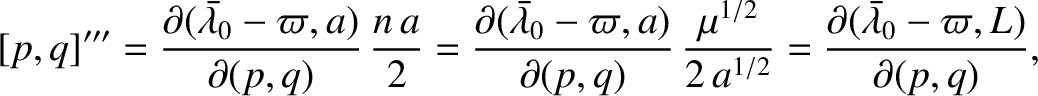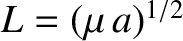(G.87) and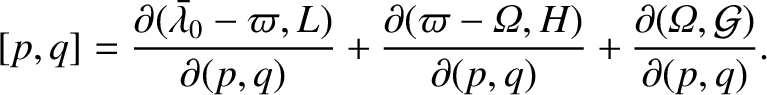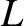(G.88)

with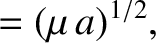. Hence,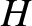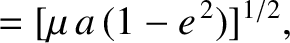(G.89)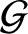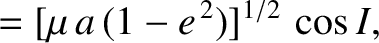(G.90)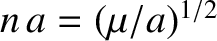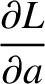(G.91)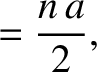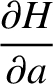(G.92)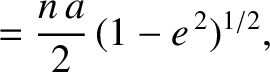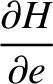(G.93) and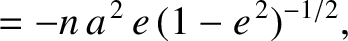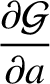(G.94)

with all other partial derivatives zero. Thus, from Equation (G.85), the only non-zero Lagrange brackets are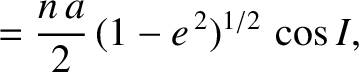(G.95)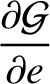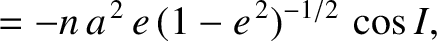(G.96)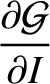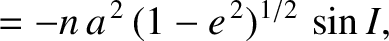(G.97)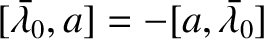(G.98)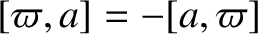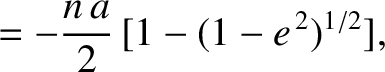(G.99) and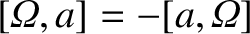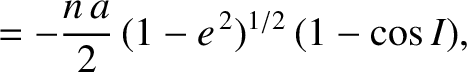(G.100)

Hence, Equations (G.32) yield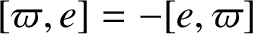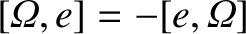(G.101)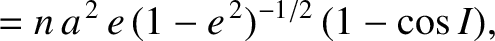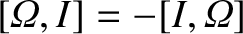(G.102)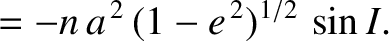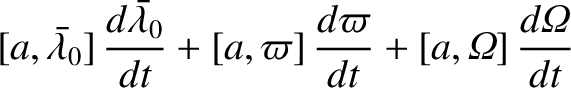(G.103)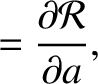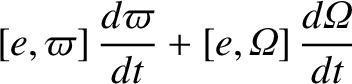(G.104)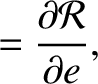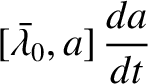(G.105) and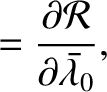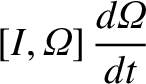(G.106)

Finally, Equations (G.95)-(G.106) can be rearranged to give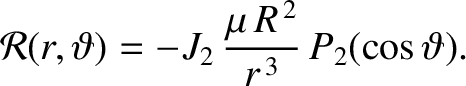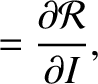(G.107)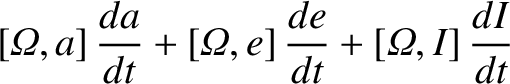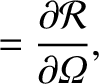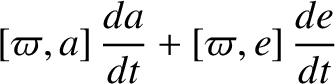(G.108)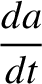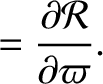(G.109)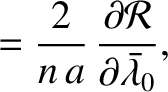(G.110)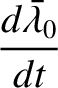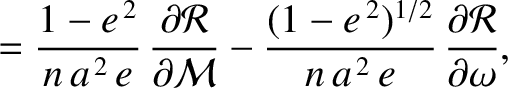(G.111) and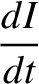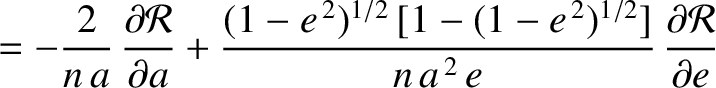(G.112)

Equations (G.107)-(G.112), which specify the time evolution of the osculating orbital elements of our planet under the action of the disturbing function, are known collectively as the Lagrange planetary equations (Brouwer and Clemence 1961).

In fact, the orbital element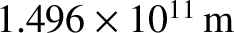always appears in the disturbing function in the combination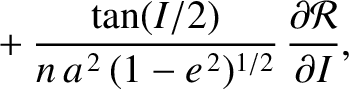. This combination is known as the mean longitude, and is denote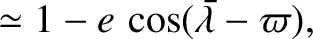. It follows that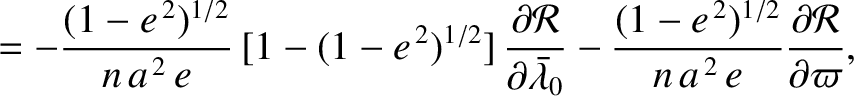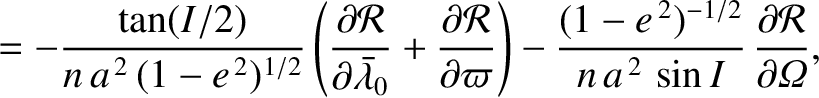(G.113) and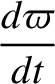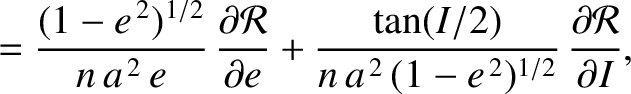(G.114)

The integral appearing in the previous equation is problematic. Fortunately, it can easily be eliminated by replacing the variableby. In this case, the Lagrange planetary equations become(G.115)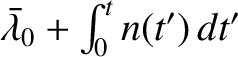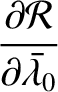(G.116)(G.117)(G.118)(G.119) and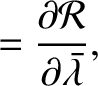(G.120)

where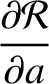is taken at constant, and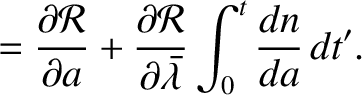at constant(Brouwer and Clemence 1961).Next: Alternative forms of Lagrange Up: Derivation of Lagrange planetary Previous: Transformation of Lagrange brackets
Richard Fitzpatrick 2016-03-31